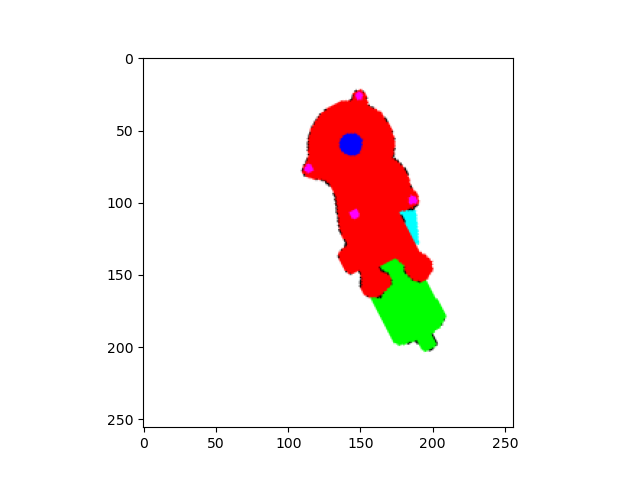# Code for mapping color codes to class indices shows non-deterministic behavior

I am working on semantic segmentation of electric motors. My masks contain 7 classes (including background) encoded as RGB colors.I use the following code which is based on this post:

``````import torch
import numpy as np
import cv2
import matplotlib.pyplot as plt

h=256
w=256

#Color codes
colors=[(255 ,0, 0),
(0,0,255),
(0,255,0),
(255,0,255),
(0,255,255),
(255,255,255),
(0,0,0)]

mapping = {tuple(c): t for c, t in zip(colors, range(len(colors)))}

target=torch.from_numpy(target)
plt.imshow(target)
plt.pause(1)
target = target.permute(2, 0, 1)

for k in mapping:
idx = (target==torch.tensor(k, dtype=torch.uint8).unsqueeze(1).unsqueeze(2))
validx = (idx.sum(0) == 3)

plt.pause(0.1)
``````

When I run the code the result should look like this:

`>>>unique values mapped tensor([0, 1, 2, 3, 4, 5, 6])`But often the mapping is messed up. For example:

``````>>>unique values mapped  tensor([                  0,                   1,                   2,
3,                   4,                   5,
6, 4575657222473777152])
``````This example is at least similar to the desired result, only a class indize was added. But there are also completely strange results with hundreds of class indices.

This happens without me changing the code or the input image. I do nothing but execute the same code again. Can someone explain to me why this happens? Where do the additional classes come from?

The `mask` tensor is initialized via `torch.empty`, which uses uninitialized memory and thus random values.
Based on the output of `torch.unique(mask)` it seems you haven’t set all values in the mask and thus the last value is coming from the uninitialized memory.

Could you check where this index is used and which color can be found there? I guess you might have missed a particular color (or it was created due to e.g. linear interpolation during resizing).

1 Like

Thank you. The issue was indeed caused by additional RGB values in the original mask. I used the ImageDataLoader from Keras for the agmentation of the masks. It seems that for some operations it changes the RGB values in the border areas. I have now solved the problem by using `torch.zeros` instead of `torch.empty`.

Is there any advantage in using `torch.empty` over `torch.zeros` in the first place? Otherwise I would use only torch.zeros in the future to avoid these kind of problems.

`torch.empty` would be faster as it doesn’t need to initialize the memory.
However, using `torch.zeros` might avoid running into this error, but you would add the “zero label” to the invalid colors, which might create invalid targets.

@ptrblck I have used the color map you suggested here but I got different outputs. The target shape is [3, 256, 256] and 21 classes but the function produces random class indices. When I use CrossEntropyLoss, this error rises up: RuntimeError: CUDA error: device-side assert triggered

def Convert_gts(n_classes, target):

``````nb_classes = n_classes - 1  # 20 classes + background
idx = np.linspace(0., 1., nb_classes)
cmap = matplotlib.cm.get_cmap('jet')
rgb = cmap(idx, bytes=True)[:, :3]  # Remove alpha value
target = target.reshape( 256 * 256, 3)
h, w = 256, 256
rgb = rgb.repeat(3276.8, 0)
target[:rgb.shape] = rgb
target = target.reshape(h, w, 3)
target = torch.from_numpy(target)
colors = torch.unique(target.view(-1, 3), dim=0).numpy()
target = target.permute(2, 0, 1).contiguous()

mapping = {tuple(c): t for c, t in zip(colors.tolist(), range(len(colors)))}

for k in mapping:
idx = (target == torch.tensor(k, dtype=torch.uint8).unsqueeze(1).unsqueeze(2))
validx = (idx.sum(0) == 3)  # Check that all channels match
``````
``````Target.Shape: torch.Size([3, 256, 256])
Colors.Shape: (21, 3)
Num_Class: 21
------------------------------------------
Target.Shape: torch.Size([3, 256, 256])
Colors.Shape: (25, 3)
Num_Class: 21
------------------------------------------
Target.Shape: torch.Size([3, 256, 256])
Colors.Shape: (27, 3)
Num_Class: 21
``````

I have used the color map you suggested here but I got different outputs. The target shape is [3, 256, 256] and has 21 classes. The function produces random class indices shape. When I use CrossEntropyLoss, this error rises up: RuntimeError: CUDA error: device-side assert triggered

``````
def Convert_gts(n_classes, target):

nb_classes = n_classes - 1  # 20 classes + background
idx = np.linspace(0., 1., nb_classes)
cmap = matplotlib.cm.get_cmap('jet')
rgb = cmap(idx, bytes=True)[:, :3]  # Remove alpha value
target = target.reshape( 256 * 256, 3)
h, w = 256, 256
rgb = rgb.repeat(3276.8, 0)
target[:rgb.shape] = rgb
target = target.reshape(h, w, 3)
target = torch.from_numpy(target)
colors = torch.unique(target.view(-1, 3), dim=0).numpy()
target = target.permute(2, 0, 1).contiguous()

mapping = {tuple(c): t for c, t in zip(colors.tolist(), range(len(colors)))}

for k in mapping:
idx = (target == torch.tensor(k, dtype=torch.uint8).unsqueeze(1).unsqueeze(2))
validx = (idx.sum(0) == 3)  # Check that all channels match

``````
``````Target.Shape: torch.Size([3, 256, 256])
Colors.Shape: (21, 3)
Num_Class: 21
`nn.CrossEntropyLoss` expects a target tensor containing class indices or in recent versions probabilities while your target tensor seems to be an RGB tensor. In case your target contains color codes you would have to map them to class indices first.